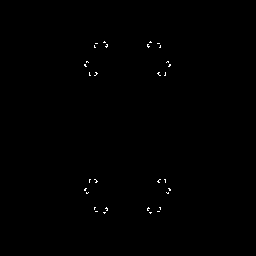There's an xscreensaver module called Julia that displays this rather more prettily. But hey, do they supply the source in Fortran-77? Sorry about using GIFs too; I know they're a dreadful format, but they work here.

# Julia Set Animation

## What it isIt's a sequence of Julia sets for the points at 5° intervals around the unit circle. It's something I wrote up in an edition of Fractal Report in the early 1990s. I animated it then in BASIC on an Amiga 500. Now, it's more accessible as a GIF animation, and the code (in an eclectic mix of Fortran77 and Perl) is presented for your "enjoyment".

Explanations of the mathematics are in Peitgen & Saupe's book The Science of Fractal Images, Springer-Verlag. They do it better than I ever could.

## How to do it

You'll need a system with Fortran, Perl, NetPBM and some kind of GIF animator. These days, cheapo PCs have quite incredible maths facilities, so you can do in a few minutes what took my old Amiga 500 all night.

First, cut out and compile the following Fortran. It really helps if you call the executable jliim3, otherwise, you'll have to redo the Perl driver.

```      program jliim3
c julia iim from "the science of fractal images"
c computes Julia sets by inverse iteration
integer width, height, maxit
parameter(width=256, height=256, maxit=100000)
integer m(width,height)

pi = 4.0 * ATAN(1.0)
hpi = pi / 2.0
x = cx
y = cy

c make array white-on-black
DO j=1,height
DO i=1,width
m(i,j)=1
END DO
END DO

DO i = 1, maxit
wx = x - cx
wy = y - cy

IF (wx.gt. 0.0) THEN
theta = ATAN(wy / wx)
ELSE
IF (wx .lt. 0) THEN
theta = pi + ATAN(wy / wx)
ELSE
theta = hpi
END IF
END IF
theta = theta / 2.0
r = SQRT(wx**2 + wy**2)
IF (rand(0.0) .lt. 0.5) THEN
r = SQRT(r)
ELSE
r = -SQRT(r)
END IF
x = r * COS(theta)
y = r * SIN(theta)
c        write(unit=*,fmt='(2f7.4)')x,y
if (i .gt. 10) then
m((width/2)+int(x*real(width/4)),
\$          (height/2)+int(y*real(height/4)))=0
c J-sets are symmetrical through the origin
m((width/2)-int(x*real(width/4)),
\$          (height/2)-int(y*real(height/4)))=0
end if
END DO

c Now write PBM file
write(unit=*,fmt='(A)')'P1'
write(unit=*,fmt=*)width,height
do j=1,height
do i=0,(width/64)-1
write(unit=*,fmt='(64I1)')(m(l,j),l=1+64*i,(64*i)+64)
end do
end do

END

c this is a free random number routine I found somewhere
function rand (r)
c r      if r=0., the next random number of the sequence is generated.
c        if r.lt.0., the last generated number will be returned for
c          possible use in a restart procedure.
c        if r.gt.0., the sequence of random numbers will start with the
c          seed r mod 1.  this seed is also returned as the value of
c          rand provided the arithmetic is done exactly.

c             output value --
c rand   a pseudo-random number between 0. and 1.

c ia1 and ia0 are the hi and lo parts of a.  ia1ma0 = ia1 - ia0.
data ia1, ia0, ia1ma0 /1536, 1029, 507/
data ic /1731/
data ix1, ix0 /0, 0/

if (r.lt.0.) go to 10
if (r.gt.0.) go to 20

c           a*x = 2**22*ia1*ix1 + 2**11*(ia1*ix1 + (ia1-ia0)*(ix0-ix1)
c                   + ia0*ix0) + ia0*ix0

iy0 = ia0*ix0
iy1 = ia1*ix1 + ia1ma0*(ix0-ix1) + iy0
iy0 = iy0 + ic
ix0 = mod (iy0, 2048)
iy1 = iy1 + (iy0-ix0)/2048
ix1 = mod (iy1, 2048)

10   rand = ix1*2048 + ix0
rand = rand / 4194304.
return

20   ix1 = amod(r,1.)*4194304. + 0.5
ix0 = mod (ix1, 2048)
ix1 = (ix1-ix0)/2048
go to 10

end
```

The above program just takes a coordinate pair on standard input, and writes a 256x256 pixel PBM file to standard output. The Perl driver (below) just calls the program many times, feeding it different coordinates, and converting the output to GIF files called j000.gif to j355.gif.

```#!/usr/bin/perl -w
# animjulia - created by scruss on Sat Jun 6 17:39:45 BST 1998

use strict;
my \$pi = 4 * atan2(1,1); # const
my \$a=0;

while (\$a < 360) {
my \$theta = \$a * \$pi / 180;
my \$x = cos(\$theta);
my \$y = sin(\$theta);
my \$f = sprintf("j%03d.gif",\$a);
system "echo \$x,\$y | jliim3 | ppmtogif -sort > \$f\n";
print STDERR \$a, "\n";
\$a += 5;
}
exit;
```

You've now got a directory-full of GIF files. Stick 'em together with your favourite animator (I used whirlgif), and groove.

You can try using coloured fractal images, but in my experience they don't look so good. They look far too determinedly trippy; that's why I use them for a video for that quality Glasgow venue, Shag (Wonder what ever happened to them?).

Stewart C. Russell, Kirkintilloch, Scotland